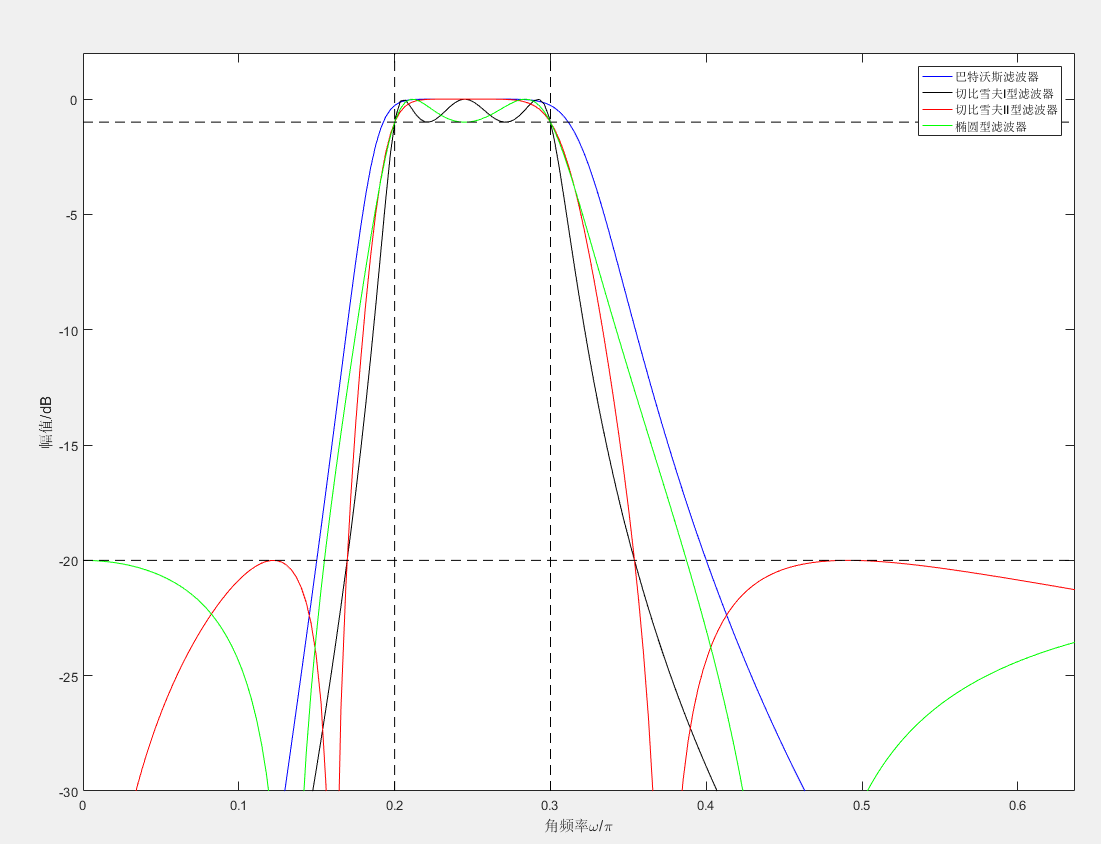• Matlab实现带通滤波器，对于研究带通采样定理很有好处的。
• 基于Matlab带通滤波器综合分析软件.pdf
• 应用切比雪夫滤波器，设计了带通滤波器 function y=bandp(x,f1,f3,fsl,fsh,rp,rs,Fs) %带通滤波 %使用注意事项：通带或阻带的截止频率与采样率的选取范围是不能超过采样率的一半 %即，f1,f3,fs1,fsh,的值小于 Fs/2 %...
盘国英谭婷的回答：你自己整合吧，我没时间帮你整合，我给你提供一些程序：绝对正确的代码：程序1：fs=22050;%语音信号采样频率为22050x1=wavread('windowscriticalstop.wav');%读取语音信号的数据，赋给变量x1sound(x1,22050);%播放语音信号y1=fft(x1,1024);%对信号做1024点fft变换f=fs*(0:511)/1024;figure(1)plot(x1)%做原始语音信号的时域图形title('原始语音信号');xlabel('timen');ylabel('fuzhin');figure(2)freqz(x1)%绘制原始语音信号的频率响应图title('频率响应图')figure(3)subplot(2,1,1);plot(abs(y1(1:512)))%做原始语音信号的fft频谱图title('原始语音信号fft频谱')subplot(2,1,2);plot(f,abs(y1(1:512)));title('原始语音信号频谱')xlabel('hz');ylabel('fuzhi');程序2：fs=22050;%语音信号采样频率为22050x1=wavread('windowscriticalstop.wav');%读取语音信号的数据，赋给变量x1t=0:1/22050:(size(x1)-1)/22050;y1=fft(x1,1024);%对信号做1024点fft变换f=fs*(0:511)/1024;x2=randn(1,length(x1));%产生一与x长度一致的随机信号sound(x2,22050);figure(1)plot(x2)%做原始语音信号的时域图形title('高斯随机噪声');xlabel('timen');ylabel('fuzhin');randn('state',0);m=randn(size(x1));x2=0.1*m+x1;sound(x2,22050);%播放加噪声后的语音信号y2=fft(x2,1024);figure(2)plot(t,x2)title('加噪后的语音信号');xlabel('timen');ylabel('fuzhin');figure(3)subplot(2,1,1);plot(f,abs(y2(1:512)));title('原始语音信号频谱');xlabel('hz');ylabel('fuzhi');subplot(2,1,2);plot(f,abs(y2(1:512)));title('加噪后的语音信号频谱');xlabel('hz');ylabel('fuzhi');根据以上代码，你可以修改下面有错误的代码程序3:双线性变换法设计butterworth滤波器fs=22050;x1=wavread('h:\课程设计2\shuzi.wav');t=0:1/22050:(size(x1)-1)/22050;au=0.03;d=[au*cos(2*pi*5000*t)]';x2=x1+d;wp=0.25*pi;ws=0.3*pi;rp=1;rs=15;fs=22050;ts=1/fs;wp1=2/ts*tan(wp/2);%将模拟指标转换成数字指标ws1=2/ts*tan(ws/2);[n,wn]=buttord(wp1,ws1,rp,rs,'s');%选择滤波器的最小阶数[z,p,k]=buttap(n);%创建butterworth模拟滤波器[bap,aap]=zp2tf(z,p,k);[b,a]=lp2lp(bap,aap,wn);[bz,az]=bilinear(b,a,fs);%用双线性变换法实现模拟滤波器到数字滤波器的转换[h,w]=freqz(bz,az);%绘制频率响应曲线figure(1)plot(w*fs/(2*pi),abs(h))gridxlabel('频率／hz')ylabel('频率响应幅度')title('butterworth')f1=filter(bz,az,x2);figure(2)subplot(2,1,1)plot(t,x2)%画出滤波前的时域图title('滤波前的时域波形');subplot(2,1,2)plot(t,f1);%画出滤波后的时域图title('滤波后的时域波形');sound(f1,22050);%播放滤波后的信号f0=fft(f1,1024);f=fs*(0:511)/1024;figure(3)y2=fft(x2,1024);subplot(2,1,1);plot(f,abs(y2(1:512)));%画出滤波前的频谱图title('滤波前的频谱')xlabel('hz');ylabel('fuzhi');subplot(2,1,2)f1=plot(f,abs(f0(1:512)));%画出滤波后的频谱图title('滤波后的频谱')xlabel('hz');ylabel('fuzhi');程序4:窗函数法设计滤波器：fs=22050;x1=wavread('h:\课程设计2\shuzi.wav');t=0:1/22050:(size(x1)-1)/22050;au=0.03;d=[au*cos(2*pi*5000*t)]';x2=x1+d;wp=0.25*pi;ws=0.3*pi;wdelta=ws-wp;n=ceil(6.6*pi/wdelta);%取整wn=(0.2+0.3)*pi/2;b=fir1(n,wn/pi,hamming(n+1));%选择窗函数，并归一化截止频率figure(1)freqz(b,1,512)f2=filter(bz,az,x2)figure(2)subplot(2,1,1)plot(t,x2)title('滤波前的时域波形');subplot(2,1,2)plot(t,f2);title('滤波后的时域波形');sound(f2,22050);%播放滤波后的语音信号f0=fft(f2,1024);f=fs*(0:511)/1024;figure(3)y2=fft(x2,1024);subplot(2,1,1);plot(f,abs(y2(1:512)));title('滤波前的频谱')xlabel('hz');ylabel('fuzhi');subplot(2,1,2)f2=plot(f,abs(f0(1:512)));title('滤波后的频谱')xlabel('hz');ylabel('fuzhi');hbchifan的回答：% 用切比雪夫最佳一致逼近设计线性相位FIR带通滤波器；%信号为0.5hz, 0.9hz, 1.1hz和1.5hz的正统信号叠加组成%通带为[0.9,1.1]%频谱分辨率与信号实际长度N成正比clear all;f1=0.5;f2=0.9;f3=1.1;f4=1.5;t=0:1203;N=length(t);fs=10;M=512;x1=sin(2*pi*(f1/fs)*t)+sin(2*pi*(f2/fs)*t)+sin(2*pi*(f3/fs)*t)+sin(2*pi*(f4/fs)*t);figure(1);subplot(211);plot(t,x1);title('原信号');y=fft(x1);f=(0:1/N:1/2-1/N)*fs;subplot(212);plot(f,abs(y(1:N/2)));grid;xlabel('hz');%处理前频谱wc1=2*f2/fs;wc2=2*f3/fs;wc3=2*f4/fs;%归一化角频率，用于下面的f1f1=[0 wc1-0.05 wc1 wc2 wc2+0.05 1];A=[0 0 1 1 0 0];%设置带通或带阻，1为带通，0为带阻weigh=[1 1 1 ];%设置通带和阻带的权重b=remez(60,f1,A,weigh);%传函分子h1=freqz(b,1,M);%幅频特性figure(2)f=(0:1/M:1-1/M)*fs/2;subplot(211);plot(f,abs(h1));grid;title('带通');x2=filter(b,1,x1);S1=fft(x2);f=(0:1/N:1/2-1/N)*fs;subplot(212);plot(f,abs(S1(1:N/2)));grid;xlabel('hz');%处理后频谱
展开全文• 原始信号由5Hz，50Hz，110Hz三种频率的正弦信号构成...​使用通带为[10,100]Hz的切比雪夫滤波器，滤波后的信号时域曲线为： ﻿图 2 滤波信号 ​对原始信号和滤波信号作傅里叶变换，观察频谱的变化（左图为原始信号...
原始信号由5Hz，50Hz，110Hz三种频率的正弦信号构成，并含有直流分量。

原始信号为：y=sin(5*2*pi*x)+sin(50*2*pi*x)+sin(110*2*pi*x)+0.5;​﻿图 1   原始信号

​使用通带为[10,100]Hz的切比雪夫滤波器，滤波后的信号时域曲线为：﻿图 2   滤波信号

​对原始信号和滤波信号作傅里叶变换，观察频谱的变化（左图为原始信号频谱，有图为滤波信号频谱）。﻿图 3   频谱对比

​对比图 3 可见，原始信号5Hz，110Hz的交流成分和直流成分都被滤掉了，只保留了50Hz交流成分，并且幅值吻合。

附切比雪夫II型带通滤波器程序

​function [X,Y]=Chebyshev2bp(x,y,fp,fs,Rp,Rs)

% fp 通带边界频率，单位Hz

% fs 阻带边界频率，单位Hz

% Rp 通带波纹，单位分贝（通常取值为1，该值越小通带幅值越平稳，但过渡带宽也越大）

% Rs 阻带衰减，单位分贝（从30附近试取，该值越大阻带幅值越小，但过渡带宽也越大）

wp=fp*2*pi;

ws=fs*2*pi;

[N,Wn]=cheb2ord(wp,ws,Rp,Rs,'s');  % 根据滤波器要求，求得滤波器最小阶数（计算也越慢）和截止频率（万永革 例5-17）

N

%%% 关于滤波器阶数N的说明

% 理论上，滤波器阶数越高越接近理想滤波器，但滤波器阶数达到一定值后再增加阶数，其逼近程度并不再显著提高，但计算量依然增加，所以理论上不需要无穷大的阶数

% 实际编程中，随着阶数无限升高，更根本的问题是计算机积累误差凸显，将使其与理想滤波器相去甚远，最终时域幅值严重失真，所以实际应用中滤波器阶数也不是越高越好

[z,p,k]=cheb2ap(N,Rs);  %设计Chebyshev II型原型低通滤波器（万永革 例5-8）

[b,a]=zp2tf(z,p,k);  % 将零点极点增益形式转换为传递函数形式

Wo=sqrt(Wn(1)*Wn(2));  % 计算中心点频率（万永革 例5-14）

Bw=Wn(2)-Wn(1);  % 计算频带宽度

[bt,at]=lp2bp(b,a,Wo,Bw);  % 频率转换。将模拟原先低通滤波器转换为带通滤波器

H=[tf(bt,at)];  % 在MATLAB中表示此滤波器（万永革 例5-13）

[Y,X]=lsim(H,y,x);

https://www.cnblogs.com/alimy/p/9140695.html
展开全文切比雪夫
• 用基本数学方程设计带通滤波器程序，对没有信号处理工具箱的人有帮助。 三角正弦函数和汉明窗方程用于创建带通滤波器。 通过改变中心频率、带宽参数，滤波器可以定制到所需的频率。 可以参考相同的程序来创建 ...
• 自己编写的小程序matlab程序带通滤波器，可设置最小截止频率和最大截止频率。 ps。不附带信号文件，很简单的原理，旨在指导带通滤波器的编程思路与原理
• 基于MATLAB仿真带通滤波器程序，包括归一化波形，频谱波形！
• 设计带通滤波器MATLAB程序.这个程序简单明了，能够很容易的进行滤波。
• matlab设计FIR 带通滤波器，实例讲解，程序代码，图像均包含。
• 最近初入信号处理，对带通滤波器的资料进行总结。 转载自： 如何在matlab带通滤波器进行滤波？ matlab中fdatool使用说明 ...自己编的一个带通滤波器程序及解释 fs=500; % 采样率 n=length(data);
最近初入信号处理，对带通滤波器的资料进行总结。
转载自：

如何在matlab用带通滤波器进行滤波？

matlab中fdatool使用说明

Matlab fir滤波(conv)

以上链接详细记录了带通滤波器的设计及matlab自带fdatool的使用步骤。
自己编的一个带通滤波器的程序及解释
fs=500;                                                 % 采样率
n=length(data);                                         % 滤波数据长度
t = 0:1/fs:(n-1)/fs;                                    % 横坐标刻度值
lpass=100;
hpass=200;                                              % 带通滤波的带宽
filterorder =2;                                         % 滤波器阶数
filtercutoff = [2*lpass/fs 2*hpass/fs];                 % 频率归一化 对应频率/nyquist频率，nyquist频率是采样率的一半
[f_b, f_a] = butter(filterorder,filtercutoff);          % 调用butter()函数返回巴特沃斯滤波器传递函数的零极点系数和增益
sig= filtfilt(f_b,f_a,data);                            % 零相位数字滤波 通过x在正向和反向两个方向上处理输入数据来执行零相位数字滤波 。在向前过滤数据后，反转过滤后的序列，然后将其运回过滤器。
noise=data-sig;                                         % 噪声为原始信号减去滤波后信号的剩余信号
SNR=10*log((sig.^2)/(noise.^2));                        % 10lg(Ps/Pn)，其中Ps和Pn分别代表信号和噪声的有效功率，也可以换算成电压幅值的比率关系：20Lg(Vs/Vn)，Vs和Vn分别代表信号和噪声电压的“有效值”。用dB表示。

有需要可以自行修改，不保证正确。


展开全文• MATLAB语言设计一个带通滤波器。给定抽样频率，通带起始频率，截止频率。阻带起始频率，截止频率。
• matlab代码，直接运行出结果。FIR带通滤波器，并对数字信号进行带通滤波。建立含有3种频率和白噪声的信号。然后利用窗函数法设计
• 这是一个数字带通滤波器程序，由模拟滤波器到数字带通滤波器
• 简单记录下在matlab上如何设计出模拟的带通滤波器，包括：巴特沃斯滤波器、切比雪夫I型滤波器、切比雪夫II型滤波器、椭圆型滤波器。 代码如下： %设计带通滤波器 %巴特沃斯、切比雪夫I型、切比雪夫II型、椭圆...


版权声明：本文为博主原创文章，如需转载，请注明出处 https://blog.csdn.net/qq_36554582/article/details/83350837

简单记录下在matlab上如何设计出模拟的带通滤波器，包括：巴特沃斯滤波器、切比雪夫I型滤波器、切比雪夫II型滤波器、椭圆型滤波器。
代码如下：

%设计带通滤波器
%巴特沃斯、切比雪夫I型、切比雪夫II型、椭圆型滤波器

clear all;

%wp和ws分别是通带和阻带的频率(截止频率)。当wp和ws为二元矢量时，为带通或带阻滤波器，这时求出的Wn也是二元矢量；当wp和ws为一元矢量时，为低通或高通滤波器：当wp<ws时为低通滤波器，当wp>ws时为高通滤波器。

%wp和ws为二元矢量
wp=[0.1*2*pi 0.15*2*pi];                %设置通带频率
ws=[0.05*2*pi 0.2*2*pi];                %设置阻带频率

Rp=1;                                   %设置通带波纹系数
Rs=20;                                  %设置阻带波纹系数

%巴特沃斯滤波器设计
[N,Wn]=buttord(wp,ws,Rp,Rs,'s');        %求巴特沃斯滤波器阶数，输出参数N代表满足设计要求的滤波器的最小阶数，Wn是等效低通滤波器的截止频率
%无论是高通、带通和带阻滤波器，在设计中最终都等效于一个截止频率为Wn的低通滤波器(我现在也不是很理解为啥是这样，毕竟我也是刚接触滤波器)
fprintf('巴特沃斯滤波器 N= %4d\n',N);    %显示滤波器阶数
[bb,ab]=butter(N,Wn,'s');               %求巴特沃斯滤波器系数，即求传输函数的分子和分母的系数向量
W=0:0.01:2;                             %设置模拟频率
[Hb,wb]=freqs(bb,ab,W);                 %求巴特沃斯滤波器频率响应
plot(wb/pi,20*log10(abs(Hb)),'b');      %作图
hold on

%切比雪夫I型滤波器设计
[N,Wn]=cheb1ord(wp,ws,Rp,Rs,'s');       %求切比雪夫I型滤波器阶数
fprintf('切比雪夫I型滤波器 N= %4d\n',N); %显示滤波器阶数
[bc1,ac1]=cheby1(N,Rp,Wn,'s');          %求切比雪夫I型滤波器系数，即求传输函数的分子和分母的系数向量
[Hc1,wc1]=freqs(bc1,ac1,W);             %求切比雪夫I型滤波器频率响应
plot(wc1/pi,20*log10(abs(Hc1)),'k');    %作图

%切比雪夫II型滤波器设计
[N,Wn]=cheb2ord(wp,ws,Rp,Rs,'s');       %求切比雪夫II型滤波器阶数
fprintf('切比雪夫II型滤波器 N= %4d\n',N);%显示滤波器阶数
[bc2,ac2]=cheby2(N,Rs,Wn,'s');          %求切比雪夫II型滤波器系数，即求传输函数的分子和分母的系数向量
[Hc2,wc2]=freqs(bc2,ac2,W);             %求切比雪夫II型滤波器频率响应
plot(wc2/pi,20*log10(abs(Hc2)),'r');    %作图

%椭圆型滤波器设计
[N,Wn]=ellipord(wp,ws,Rp,Rs,'s');       %求椭圆型滤波器阶数
fprintf('椭圆型滤波器 N= %4d\n',N);      %显示滤波器阶数
[be,ae]=ellip(N,Rp,Rs,Wn,'s');          %求椭圆型滤波器系数，即求传输函数的分子和分母的系数向量
[He,we]=freqs(be,ae,W);                 %求椭圆型滤波器频率响应
%作图
plot(we/pi,20*log10(abs(He)),'g');
axis([0 max(we/pi) -30 2]);
legend('巴特沃斯滤波器','切比雪夫I型滤波器','切比雪夫II型滤波器','椭圆型滤波器');
xlabel('角频率{\omega}/{\pi}');
ylabel('幅值/dB');
line([0 max(we/pi)],[-20 -20],'color','k','linestyle','--');%在画布上画线
line([0 max(we/pi)],[-1 -1],'color','k','linestyle','--');
line([0.2 0.2],[-30 2],'color','k','linestyle','--');
line([0.3 0.3],[-30 2],'color','k','linestyle','--');


运行结果如下：

巴特沃斯滤波器 N=    4
切比雪夫I型滤波器 N=    3
切比雪夫II型滤波器 N=    3
椭圆型滤波器 N=    2注：
1、求各种滤波器的传输函数的分子和分母向量系数的函数，例如：

[bc1,ac1]=cheby1(N,Rp,Wn,'s');          %求切比雪夫I型滤波器系数，即求传输函数的分子和分母的系数向量


这里的函数cheby1()中的参数为

cheby1(N,Rp,Wn,'type','s');


其中注意’type’可为高通：'high’或带阻：‘stop’，如果不填的话，默认是低通或者带通，至于是低通还是带通，主要看你前面的wp和ws是二元矢量还是一元矢量：二元矢量对应带通，一元矢量对应低通。

2、matlab中的line()函数简单介绍：https://blog.csdn.net/qq_36554582/article/details/83352155

展开全文• 基于MATLAB的FIR带通滤波器的设计与仿真 摘要利用数字信号处理理论与方法基于MATLAB语言通过实例设计FIR数字带通滤波器给出了MATLAB完整程序实例仿真结果表明该带通滤波器的滤波效果达到了预期目的 关键词数字滤波器...
• 产生3个正弦成份（15hz 55hz 75hz）的信号 用带通滤波器去除15和75hz 成份。
• Butterworth巴特沃斯带通滤波器音频分离Matlab程序，FFT频域处理，音频分离。
• 看到一个带通滤波器的设计如下，开始研究是如何设计的 代码： band_low = 0.3; band_high = 1.2; fs_origin = 25; hd_band = design(fdesign.bandpass('N,F3dB1,F3dB2',4,band_low,band_high,fs_origin),'...
• Matlab通过创建应用程序M文件为GUI控制程序提供一个框架。所有代码(包括回调函数)都包含在应用程序M文件，即完成了图形界面的设计后，应用程序设计的主要工作是控件回调函数的设计。下面讨论程序设计流程及控件回...
• 自学应用程序或大学实验室，让学生通过实际实验找出以下问题的答案：a) 放置极点和​​零点 b) 设计的 IIR 带通滤波器之间的截止锐度和稳定性有何不同) 使用双线性变换 (BLT)？ 可以选择不同的滤波器类型并听到...
• 程序利用matlab实现等波纹最佳逼近法设计带通滤波器，内含实例代码。
• ## 死磕带通滤波器

千次阅读 2021-04-24 20:51:17
带通滤波器的作用 与陷波器类似，带通滤波器在数字电源控制领域有重要作用。比如在三相LCL逆变器的谐振抑制控制方面，通过带通滤波器可以提取谐振点附近的频谱做进一步的控制策略。在有源电力滤波器利用带通滤波器...
• matlab 双线性变换法设计 用切比雪夫法 带通滤波器程序代码是我做课程设计时做的，可以出来比较理想的结果
• 与我齐飞巴特沃斯：1、带阻滤波器设计带阻滤波器指标：阻带上边界频率：5Kz；阻带下边界频率：7Kz；通带上边界频率：2Kz；通带下边界频率：9Kz；通带最大衰减：1dB；阻带最小衰减：20dB；设计程序如下：wp=2*pi*...
• 二阶有源带通滤波器设计1、背景对于微弱的信号的处理方式一般是：放大和滤波，这个过程就涉及到放大电路的选取、滤波器的选择以及偏置电路的设计。本例以实例的方式讲解并附带参数计算、仿真、实物测试三个环节。 ...
• 巴特沃斯带通滤波器matlab代码肌电图 注意：这是我多年未曾使用过的学校项目的旧代码，太幼稚了。 使用面向对象的方法会更好。 信号处理位是声音，因此请使用它们作为灵感，但我不建议在此使用任何其他功能。 ......

# matlab中带通滤波器程序matlab 订阅